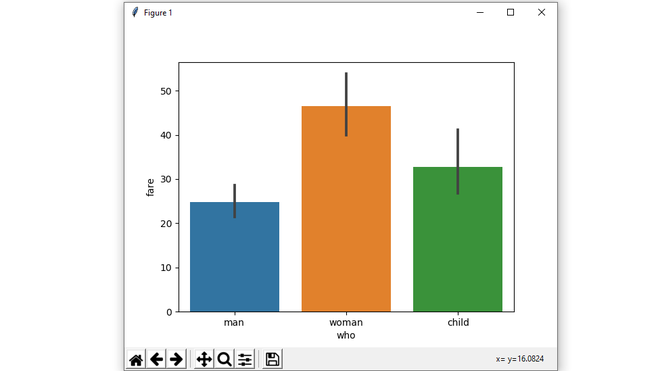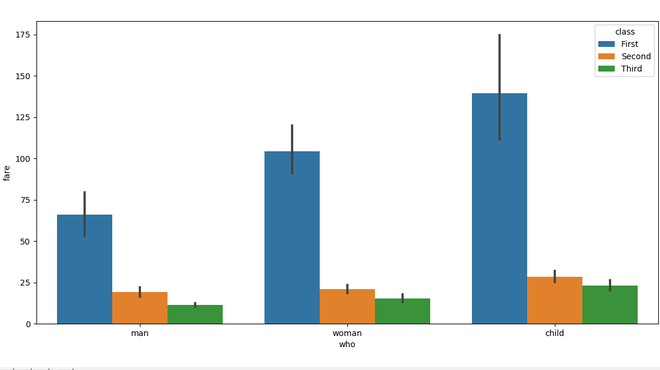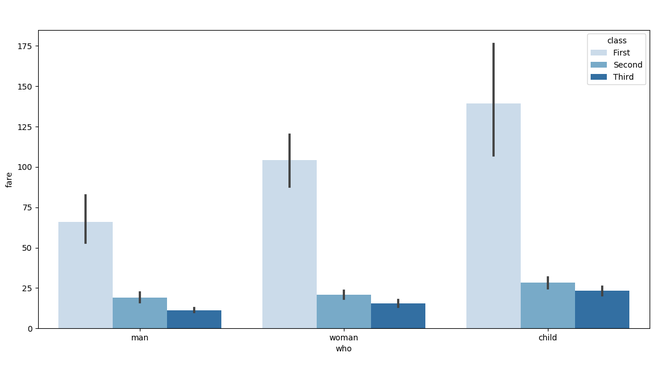Related Articles
Seaborn.barplot() method in Python
• Last Updated : 10 Aug, 2020

Seaborn is a Python data visualization library based on Matplotlib. It provides a high-level interface for drawing attractive and informative statistical graphics. There is just something extraordinary about a well-designed visualization. The colors stand out, the layers blend nicely together, the contours flow throughout, and the overall package not only has a nice aesthetic quality, but it provides meaningful insights to us as well.

## seaborn.barplot() method

A barplot is basically used to aggregate the categorical data according to some methods and by default it’s the mean. It can also be understood as a visualization of the group by action. To use this plot we choose a categorical column for the x-axis and a numerical column for the y-axis, and we see that it creates a plot taking a mean per categorical column.

Syntax : seaborn.barplot(x=None, y=None, hue=None, data=None, order=None, hue_order=None, estimator=<function mean at 0x000002BC3EB5C4C8>, ci=95, n_boot=1000, units=None, orient=None, color=None, palette=None, saturation=0.75, errcolor=’.26′, errwidth=None, capsize=None, dodge=True, ax=None, **kwargs,)

Parameters :

Following steps are used :

• Import Seaborn
• Load Dataset from Seaborn as it contain good collection of datasets.
• Plot Bar graph using seaborn.barplot() method.

Below is the implementation :

Example 1:

## Python3

 `# importing the required library``import` `seaborn as sns``import` `matplotlib.pyplot as plt` `# read a titanic.csv file``# from seaborn libraray``df ``=` `sns.load_dataset(``'titanic'``)` `# who v/s fare barplot``sns.barplot(x ``=` `'who'``,``            ``y ``=` `'fare'``,``            ``data ``=` `df)` `# Show the plot``plt.show()`

Output:Example 2:

## Python3

 `# importing the required library``import` `seaborn as sns``import` `matplotlib.pyplot as plt` `# read a titanic.csv file``# from seaborn libraray``df ``=` `sns.load_dataset(``'titanic'``)`  `# who v/s fare barplot``sns.barplot(x ``=` `'who'``,``            ``y ``=` `'fare'``,``            ``hue ``=` `'class'``,``            ``data ``=` `df)` `# Show the plot``plt.show()`

Output:Example 3:

## Python3

 `# importing the required library``import` `seaborn as sns``import` `matplotlib.pyplot as plt` `# read a titanic.csv file``# from seaborn libraray``df ``=` `sns.load_dataset(``'titanic'``)`  `# who v/s fare barplot``sns.barplot(x ``=` `'who'``,``            ``y ``=` `'fare'``,``            ``hue ``=` `'class'``,``            ``data ``=` `df,``            ``palette ``=` `"Blues"``)` `# Show the plot``plt.show()`

Output:Example 4:

## Python3

 `# importing the required library``import` `seaborn as sns``import` `matplotlib.pyplot as plt``import` `numpy as np` `# read a titanic.csv file``# from seaborn libraray``df ``=` `sns.load_dataset(``'titanic'``)`  `# who v/s fare barplot``sns.barplot(x ``=` `'who'``,``            ``y ``=` `'fare'``,``            ``hue ``=` `'class'``,``            ``data ``=` `df,``            ``estimator ``=` `np.median,``            ``ci ``=` `0``)` `# Show the plot``plt.show()`

Output:Attention geek! Strengthen your foundations with the Python Programming Foundation Course and learn the basics.

To begin with, your interview preparations Enhance your Data Structures concepts with the Python DS Course.

My Personal Notes arrow_drop_up